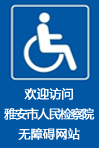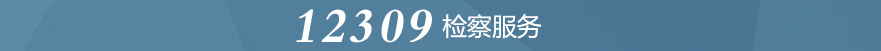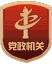• []
• []
• []
• []
• []
• []
• []

5月26日，县检察院党组书记、检察长吴超平如期到石棉县信访接待中心接待群众来访。
• []
• []
• []
• []
• []
• []
• []

• []
• []
• []
• []
• []
• []
• []

• []
• []
• []
• []
• []
• []
• []• []
• []
• []
• []
• []
• []
• []

• []
• []
• []
• []
• []
• []
• []